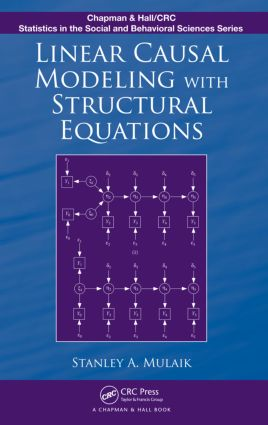Linear Causal Modeling with Structural Equations

1st Edition

Chapman and Hall/CRC

468 pages | 96 B/W Illus.

Purchasing Options:\$ = USD
Hardback: 9781439800386
pub: 2009-06-16
SAVE ~\$21.00
\$105.00
\$84.00
x
eBook (VitalSource) : 9780429133572
pub: 2009-06-16
from \$49.48

FREE Standard Shipping!

Description

Emphasizing causation as a functional relationship between variables that describe objects, Linear Causal Modeling with Structural Equations integrates a general philosophical theory of causation with structural equation modeling (SEM) that concerns the special case of linear causal relations. In addition to describing how the functional relation concept may be generalized to treat probabilistic causation, the book reviews historical treatments of causation and explores recent developments in experimental psychology on studies of the perception of causation. It looks at how to perceive causal relations directly by perceiving quantities in magnitudes and motions of causes that are conserved in the effects of causal exchanges.

The author surveys the basic concepts of graph theory useful in the formulation of structural models. Focusing on SEM, he shows how to write a set of structural equations corresponding to the path diagram, describes two ways of computing variances and covariances of variables in a structural equation model, and introduces matrix equations for the general structural equation model. The text then discusses the problem of identifying a model, parameter estimation, issues involved in designing structural equation models, the application of confirmatory factor analysis, equivalent models, the use of instrumental variables to resolve issues of causal direction and mediated causation, longitudinal modeling, and nonrecursive models with loops. It also evaluates models on several dimensions and examines the polychoric and polyserial correlation coefficients and their derivation.

Covering the fundamentals of algebra and the history of causality, this book provides a solid understanding of causation, linear causal modeling, and SEM. It takes readers through the process of identifying, estimating, analyzing, and evaluating a range of models.

Reviews

"…an accessible yet rigorous treatment of the subject and is likely to be appealing to a wide statistical audience. … I enjoyed reading this book and suspect others will too. I would recommend this book for students and researchers [who] are familiar with standard applied statistics and causal inference but are looking for an introduction to structural equation modeling."

—Eric Laber, Journal of the American Statistical Association, September 2013

"The book is written by one of the most prominent researchers in the field of structural equation models (SEM). … It is primarily a useful textbook for graduate students but could also be very useful for researchers in quantitative methods. … the book presents the standard methods of SEM in a form that makes them interesting to students and researchers with interests in the philosophical treatment of causality using SEM. … a very useful textbook for graduate students. It stands out for its rigorous treatment of SEM as a whole and for a particularly useful philosophical treatment of causality. … Stanley Mulaik’s book is one of the most useful ones with which to start a journey in this field."

—Spiridon Penev, Australian & New Zealand Journal of Statistics, 2011

"The book benefits very substantially from the author’s mixed background in multivariate analysis, psychometrics, and philosophy of science—a background which is ideally suited to the eclectic issues raised by considerations of causality. I am sure the volume will prove to be a very useful contribution to the literature, an excellent text for someone intending to research in this area, and a useful reference source for those already doing so."

—David J. Hand, International Statistical Review (2011), 79

Introduction

The Rise of Structural Equation Modeling

An Example of Structural Equation Modeling

Mathematical Foundations for Structural Equation Modeling

Introduction

Scalar Algebra

Vectors

Matrix Algebra

Determinants

Treatment of Variables as Vectors

Maxima and Minima of Functions

Causation

Historical Background

Perception of Causation

Causality

Conditions for Causal Inference

Nonlinear Causation

Science as Knowledge of Objects Demands Testing of Causal Hypotheses

Summary and Conclusion

Graph Theory for Causal Modeling

Directed Acyclic Graphs

Structural Equation Models

Basics of Structural Equation Models

Path Diagrams

From Path Diagrams to Structural Equations

Formulas for Variances and Covariances in Structural Equation Models

Matrix Equations

Identification

Incompletely Specified Models

Identification

Estimation of Parameters

Discrepancy Functions

Derivatives of Elements of Matrices

Parameter Estimation Algorithms

Designing SEM Studies

Preliminary Considerations

Multiple Indicators

The Four-Step Procedure

Testing Invariance across Groups of Subjects

Modeling Mean Structures

Confirmatory Factor Analysis

Introduction

Early Attempts at Confirmatory Factor Analysis

An Example of Confirmatory Factor Analysis

Faceted Classification Designs

Multirater-Multioccasion Studies

Multitrait-Multimethod Covariance Matrices

Equivalent Models

Introduction

Definition of Equivalent Models

Replacement Rule

Equivalent Models That Do Not Fit Every Covariance Matrix

A Conjecture about Avoiding Equivalent Models by Specifying Nonzero Parameters

Instrumental Variables

Introduction

Instrumental Variables and Mediated Causation

Conclusion

Multilevel Models

Introduction

Multilevel Factor Analysis on Two Levels

Multilevel Path Analysis

Longitudinal Models

Introduction

Simplex Models

Latent Curve Models

Reality or Just Saving Appearances?

Nonrecursive Models

Introduction

Flow Graph Analysis

Mason’s Direct Rule

Covariances and Correlations with Nonrecursive-Related Variables

Identification

Estimation

Applications

Model Evaluation

Introduction

Errors of Fit

Chi-Square Test of Fit

Properties of Chi-Square and Noncentral Chi-Square

Goodness-of-Fit Indices, CFI, and Others

The Meaning of Degrees of Freedom

"Badness-of-Fit" Indices, RMSEA, and ER

Parsimony

Information Theoretic Measures of Model Discrepancy

AIC Does Not Correct for Parsimony

Is the Noncentral Chi-Square Distribution Appropriate?

BIC

Cross-Validation Index

Confusion of "Likelihoods" in the AIC

Other Information Theoretic Indices, ICOMP

LM, WALD, and LR Tests

Modifying Models Post hoc

Recent Developments

Criticisms of Indices of Approximation

Conclusion

Polychoric Correlation and Polyserial Correlation

Introduction

Polychoric Correlation

Polyserial Correlation

Evaluation

References

Index